# Floating Point Error Handling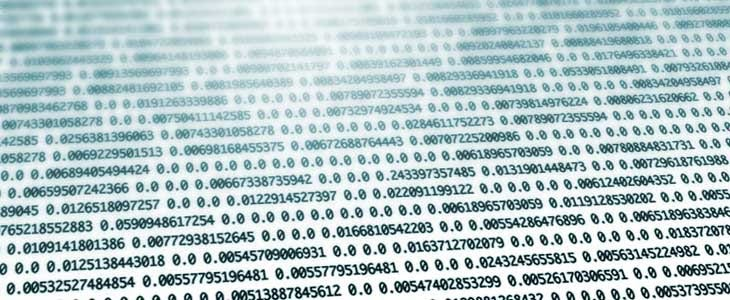Photo By Google

In this blog, I going to explain how computers generate an error when we using the floating point, and how to handle that error by using the BigDecimal.

If you don’t understand about floating point IEEE-754 standard representation in java check my previous blog.

Refer:

let’s take an example code to explain the error;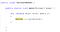Example Code

Above the for-loop will generate a result as not equal to “0” and it's executing as an infinity loop.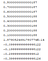The output of that Example

If using the “break” keyword to make the finite loop, the results not given as “0”.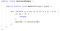Using break Keyword for that example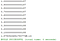Output for that example which is used the “break” Keyword

It is never will equal to zero, So how to solve this problem?

# BigDecimal in Java

It’s a predefined class since (JDK 1.5) having some great functionality. It belongs to java.math.BigDecimal class.

This class provides operations on double numbers for rounding, scale manipulation, hashing, and some other purpose. It handles the very large and very small floating point numbers with great precision.

Big decimal provides exact answers. It has provided some methods for arithmetic, logical operations as for arithmetic operations it has generated methods like add(), subtract(), divide(), and multiply().

For logical operations it has given compareTo() method which returns;

( -1 ) if the value is less than the given value

0 if it equals and 1 if the value is greater than the value.

These operations can handle the decimal values using a scale() and round() methods.

Let’s take the example to view how the BigDecimal solve the rounding issues on the computer;

Once execute this code by using this BigDecimal it will get the rounding value in every output and the execution will stop when it comes to “0”. The output of that code is below.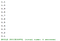The output of that code by using BigDecimal

So in java, These kinds of Floating point issues can be sorted by using the BigDecimal class.

References:

Software Engineer at Virtusa (Pvt) Ltd.

## More from Arun prashanth

Software Engineer at Virtusa (Pvt) Ltd.